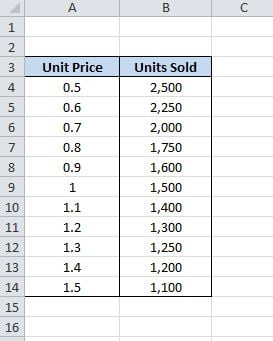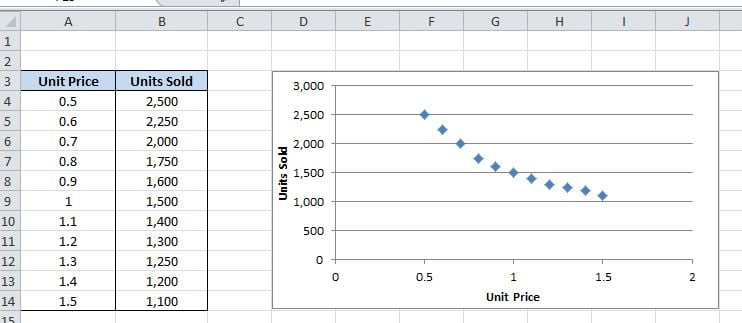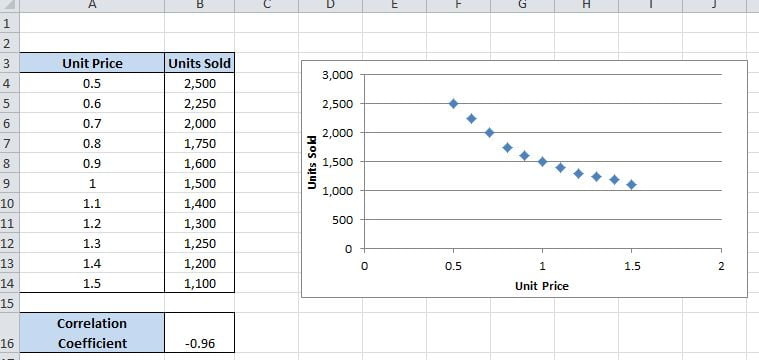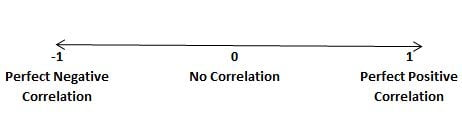# How to Calculate Correlation Coefficients in Excel 2010?

In a business environment, the Excel analyst will often be asked to prove theories and test concepts, one of the common theories to test is whether there is any correlation (or relationship) between 2 variables, for example, is there a correlation between the price of our product and a number of units we sale?

Correlation in Excel

Excel makes it quite simple to perform a correlation test, once you know what you are doing, but first of all, let’s make it clear what we are looking at in this article as it is important.

Assumption

The key assumption is that your data is linear. Linear data takes the form of such things as price, volume, height, weight etc. There is no upper or lower limit on linear data (although your data may have min and max points). Non-Linear, for example, would be something like a location, which will always have set categories and that requires a different technique.

Example

In the following spreadsheet we have 2 variables, Unit Price (Column A) and Units Sold (Column B), from this, we want to test if there is any correlation between the two:One of the first steps you should do is create a Scatter Chart of the data.  This is not totally necessary but is good practice as visualising the data can help us understand what is happening.  To create a quick Scatter Chart highlight the data, then left-click on “Insert” on the top icon list, followed by “Scatter” to select your chart:We will now have a worksheet looking something like this:Now straight away you can see that the as price is going up the volume is coming down, it looks like there is a correlation between the two variables, but we still need to prove this and find out how correlated the two variables are.

The next step is to get Excel to calculate the Correlation Coefficient for the two variables, the correlation coefficient will tell us exactly how strong the relationship between unit price and units sold is.

CORREL Function

To calculate the correlation coefficient in Excel we use the CORREL function, which takes the format:

=CORREL(Array1, Array2)

This is where Array1 and Array2 are the cell ranges of your data.

Applied to our example we would select an empty cell and use the formula:

=CORREL(A4:A14, B4:B14)The result of our calculation is -0.96 (rounded to 2 decimal places):Interpreting the Result

Now that we have got Excel to calculate the correlation coefficient we need to interpret it to understand its true meaning.Â  The value is always between -1 and +1 and the following scale shows how to interpret:A value of zero tells us that there is no correlation between our 2 variables, a value of positive 1 tells us there is perfect positive correlation, which means as one variable goes up so does the other and finally a value of negative 1 tells us we have perfect negative correlation, as one variable goes up the other goes down.

It is rare to find perfect correlation (+1 or -1) so depending how close your result is on the scale you must adjust your conclusions, a commonly used scale is:

 Positive Negative No Correlation 0 to 0.1 0 to -0.1 Weak Correlation exists 0.1 to 0.3 -0.1 to -0.3 Medium Correlation exists 0.3 to 0.6 -0.3 to -0.6 Strong Correlation exists 0.6 to 1 -0.6 to -1

For our example we calculated a Correlation Coefficient of -0.96. Looking at the scale this tells us we have strong negative correlation in the data.

What this means in plain-English is that as Unit Price increases Units sold decreased. The correlation coefficient suggests that this is a strong correlation so we can be confident this is always the case for that product. If we had produced a result of -0.2 then we would change our conclusion to say “weak negative correlation exists but we can’t be totally confident this will always be true”.

Summary

I hope that this has proved a useful exercise to you. There are other statistical tests to calculate correlation between non-linear data but that will be in a future post. As long as both your variables are linear data then this is the correct technique to apply, simple yet very effective once you know how.

Happy Excelling
Team Excelgoodies
www.Excelgoodies.com